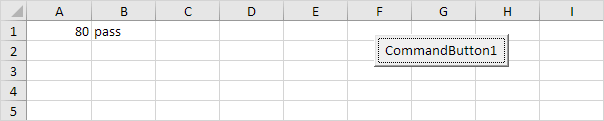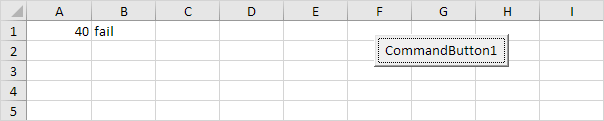# If Then Statement

Use the If Then statement in Excel VBA to execute code lines if a specific condition is met.

### If Then Statement

Place a command button on your worksheet and add the following code lines:

Dim score As Integer, result As String
score = Range("A1").Value

If score >= 60 Then result = "pass"

Range("B1").Value = result

Explanation: if score is greater than or equal to 60, Excel VBA returns pass.

Result when you click the command button on the sheet:Note: if score is less than 60, Excel VBA places the value of the empty variable result into cell B1.

### Else Statement

Place a command button on your worksheet and add the following code lines:

Dim score As Integer, result As String
score = Range("A1").Value

If score >= 60 Then
result = "pass"
Else
result = "fail"
End If

Range("B1").Value = result

Explanation: if score is greater than or equal to 60, Excel VBA returns pass, else Excel VBA returns fail.

Result when you click the command button on the sheet:Note: only if you have one code line after Then and no Else statement, it is allowed to place a code line directly after Then and to omit (leave out) End If (first example). Otherwise start a new line after the words Then and Else and end with End If (second example).

Go to Next Chapter: Loop